# Funky definitions of prime number

## Definitions in terms of arithmetic functions

### Divisor count function

A natural numberis prime if and only ifwhere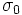is the divisor count function.

### Divisor sum function

A natural numberis prime if and only if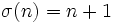, where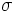is the divisor sum function.

### Divisor power sum function

For any, a natural numberis prime if and only if, where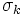is thedivisor power sum function.

### Euler phi-function

A natural numberis prime if and only if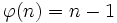, whereis the Euler phi-function.

### Dedekind psi-function

A natural numberis prime if and only if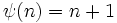, whereis the Dedekind psi-function.

### von Mangoldt function

A natural numberis prime if and only ifand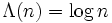whereis the von Mangoldt function.

## In terms of facts true for prime numbers

### Wilson's theorem

A natural numberis prime if and only ifand.

## In algebraic terms

### Groups

A natural numberis prime if and only if it satisfies the following equivalent conditions:

• The subgroupis a maximal subgroup in the group of integers.
• The group of integers modulois a simple group.

### Rings and fields

A nautral numberis prime if and only if it satisfies the following equivalent conditions:

• The idealis a maximal ideal in the ring of integers.
• The ring of integers modulois a field.

## In terms of primality tests

Fill this in later